## Saturday, May 21, 2011

### F1 2011 Spain Free Practice - Laptime Distributions

A visual summary of laptime distributions

(Note that laptimes exceeding 1.5 times the time of a driver's fastest lap are not shown.)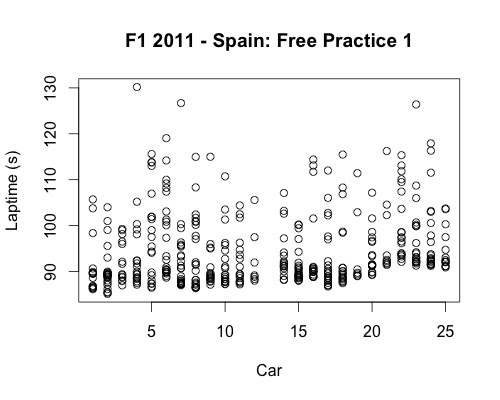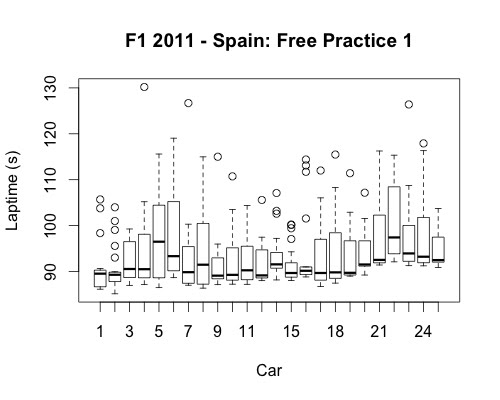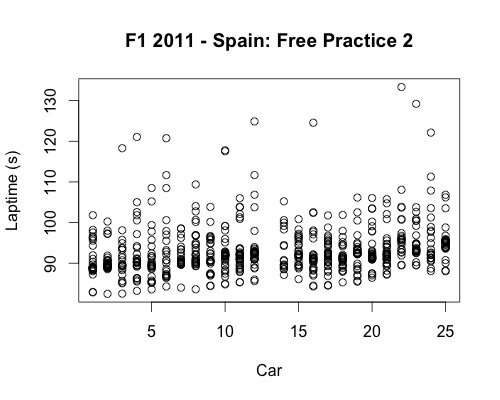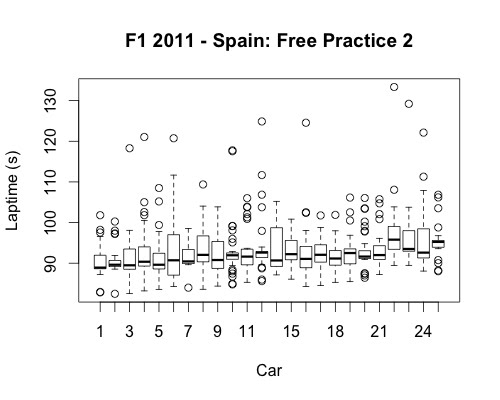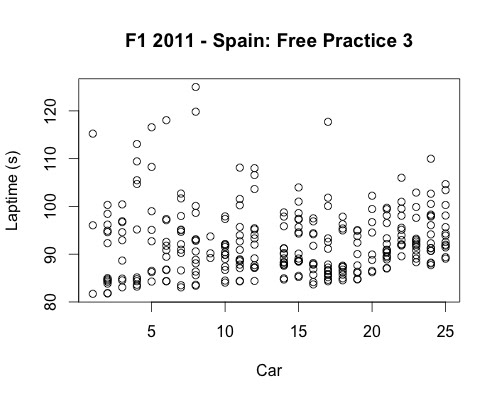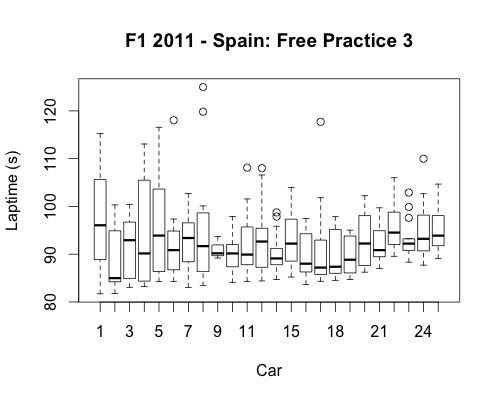The R commands used to generate these images are of the form:

plot(Time ~ DriverNum,data=esp_2011p3laptimes, main='F1 2011 - Spain: Free Practice 3',xlab="Car",ylab="Laptime (s)")

and

boxplot(Time ~ DriverNum,data=esp_2011p3laptimes, main='F1 2011 - Spain: Free Practice 3',xlab="Car",ylab="Laptime (s)")

PS here's a better R recipe for plotting charts with driver name labels:

//set font size
par(ps=15)
plot(Time ~ DriverNum,data=esp_2011p3laptimes, main='F1 2011 - Spain: Free Practice 3',xlab="Driver",ylab="Laptime (s)",xaxt='n')
labels=c("VET","WEB","HAM","BUT","ALO","MAS","SCH","ROS","HEI","PET","BAR","MAL","","SUT","RES","KOB","PER","BUE","ALG","TRU","KOV","KAR","LIU","GLO","AMB")
//set font size
par(ps=10)